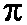## Teaching at OSU

• Winter 04: MATH 152 (Calculus 2)
• Autumn 03: MATH 150 (PreCalculus)
• Spring 03: MATH 512 (Partial Differential Equations and Boundary Value Problems)
• Winter 03: MATH 151 (Calculus I)
• Autumn 02: MATH 415 (Ordinary and Partial Differential Equations)

Cumulative Evaluation of my teaching at OSU (points are out of 5)

## Teaching at NYU

• Summer 02: Intro to Probability
• Spring 02: Advanced Calculus II
• Spring 01: Discrete Mathematics
• Fall 00: Calculus I
• Spring 00: TAed for Advanced Calculus II (taught some of the lectures, and ran problem sessions)
• Fall 99: Ran a workshop for the Written Comprehensive Exams
• Spring 99: Ran lab sessions for Quantitative Reasoning
• Fall 98: Ran problem sessions for Graduate Complex Variables
• Summer 98: Calculus I
• Spring 98: Mathematical Thinking
• Fall 97: Gave recitation sections for PreCalculus